Currently there is no translation for this activity.

# Can you find the exoplanet?

Created: 2023-11-13
Author(s):
Roger Ferlet (F-HOU, IAP), Anne-Laure Melchior (F-HOU, LERMA / Observatoire de Paris), Emmanuel Rollinde (F-HOU, LDAR / CY Cergy Paris Université)

In this activity you will learn how to reveal the presence of a planet orbiting a star other than the Sun using the “transit method”. You will use an open source software to observe a time series of images of the sky taken by the Spitzer Space Infrared Telescope, and to measure the change in light intensity of three different stars over time. You will then observe which one has a variation over a short period and understand that this decrease corresponds to the transit of a planet along the star's line of sight. Using a simplified model of the planet/star system, you will also be able to determine the planet's radius from your observations.Materials
• A file with more information on the normalisation process for the teacher's knowledge (optional): normalisation.pdfGoals
• Learning what exoplanets are
• Understanding that astronomical images are data and that you can make measurements with themLearning Objectives

Students will learn:

• What are exoplanets and how we detect them with the transit method
• Properties of an astronomical image and measurement (pixel, signal intensity)
• Creating a curve on a spreadsheet
• How graphs work and how to differentiate between the information presented in abscissa and ordinate.Background

Extrasolar Planets

An extrasolar planet, or exoplanet, is a planet outside the Solar System, orbiting a star other than the Sun. Exoplanets are very far from Earth, and they do not shine their own light but only reflect the light from the star. One can only very rarely distinguish the planets from the star in one image, as the angular separation is much lower than the halo of light received. Yet, scientists have understood how they may prove the existence of an exoplanet.

One strategy is the “radial velocity method”, based on the observation of the movement of the star itself due to its interaction with the planet. This method led to the discovery of the first exoplanet in 1995 by Michel Mayor and Didier Queloz (Observatoire de Paris, France).

Another method to detect exoplanets is called the “transit” method. It is based on the decrease in the amount of light we receive from the star due to the passage of the planet in the line of sight between the star itself and Earth. It is similar to an eclipse: when the moon passes between Earth and the Sun, it is “almost” dark, and when Venus moves between Earth and the Sun, one may observe a black disk on the Sun that blocks a few photons. The further away from Earth the object is, the smaller the occultation of the Sun. Hence, no black disk can be seen for an exoplanet. Yet, some photons are still blocked by the planet, and the bigger the planet is, the more photons are blocked. This method was proposed long ago, in 1852! (see references for historical texts). However, it requires very large and powerful telescopes, and it was only in 2004 that it first enabled the discovery of a planet.

Since 2010, hundreds of exoplanets have been discovered every year (5400 as of June 2023)! If you want to know all about exoplanets, visit the website http://exoplanet.eu and try out the graphs and diagrams.

The exoplanet orbiting star HD189733

The exoplanet you will discover in this activity orbits the star HD189733, located about 63 light-years from the Sun in the constellation Little Fox, near the constellation Cygnus in the Northern Hemisphere. The exoplanet was discovered in France on 5 October 2005 and is a "hot Jupiter" exoplanet type, meaning it has a mass comparable to that of Jupiter. However, it is closer to its star than Mercury is to the Sun, completing one orbit in just 2.2 days.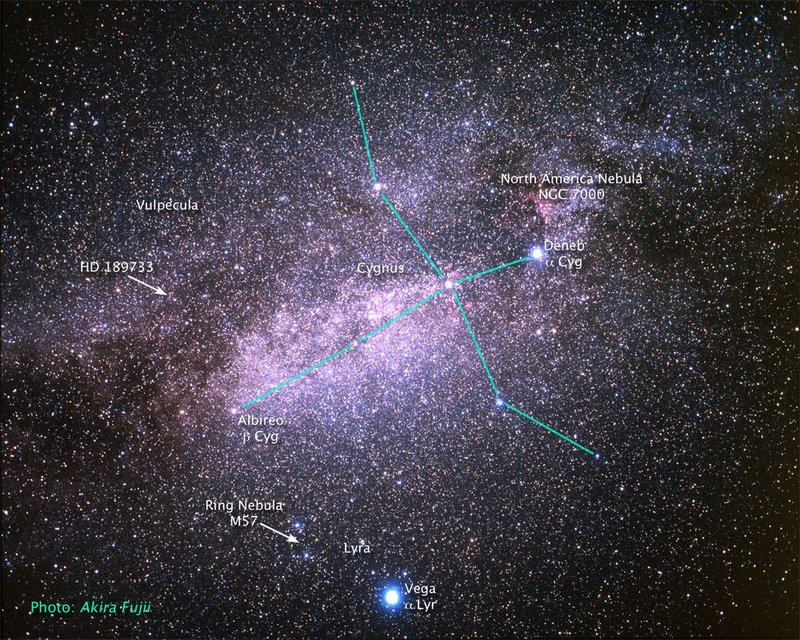Image: HD 189733 location. Credit Akira Fujii and Zolt Levay

To know more: https://en.wikipedia.org/wiki/HD_189733

How can the transit method give information about the planet?

To discover the exoplanet orbiting around star HD189733, students will use the transit method: they will observe a series of images of a small portion of the sky, taken at 13-minute intervals by the Spitzer Space Infrared Telescope, launched by NASA in 2003. These images show three stars and students will measure their change in light intensity over time (called light curve), looking for a variation over a short period. This variation of light corresponds to the decrease in the number of photons emitted by the star and captured on Earth as the planet passes along the star's line of sight, indicating the presence of an exoplanet as shown in the image below.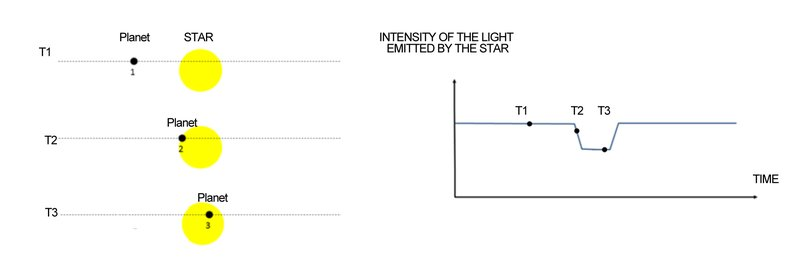Image: Representation of a planet orbiting its star at 3 different moments (T1, T2 and T3), and the intensity of the light received on Earth when observing the star.

It is important to understand that the intensity of light observed is related to the number of photons the telescope receives. This is in turn related to the surface of the star, and when a planet transits in front of the star as in the image above, some photons will be blocked by the planet. Note that, since the star is so far away, the paths of the photons from the star to the telescope can be considered parallel. Thus, there is no projection effect and the occulting surface is equal to the surface of the planet.

Using a simplified model of the planet/star system, we can say the following.

Let r and R be the radii and s and S the areas of the planet and the star, respectively. We also assume that the emission of light is about the same all over the surface of the star. Hence, when the planet is not between Earth and the star (as in T1), the intensity is proportional to the surface S:

I_0 = K * S.

When the planet is between the Star and Earth (as it is in T2 and T3), the photons blocked come from an area of surface s. Then the intensity is:
I_1 = K * (S-s).

The ratio I_1 / I_0 is equal to 1-s/S during the transit, with s/S=r^2/R^2

The parameters of stars such as mass, volume and age can be calculated from the stars' spectra, making it possible to determine those of the planet.Full Description

## Before starting the activity

Students will use SalsaJ® software, that they will have to install on a computer. It is recommended to install the software on the desktop of the computer, downloading the folder on the desktop of your computer (you can find the Link in List of material). The images are already included in the installation folder

Note 1: The analyses that will be carried out by the students are those that have been done by researchers. The complete research work includes a second method based on the radial velocity method and an in-depth work on the estimation of uncertainties. The results of this research are described in an article in English:
https://www.aanda.org/articles/aa/abs/2005/46/aahi291/aahi291.html

Note 2: It is possible to distribute the measurements across the whole class so that the work done by students is less repetitive. However, it is useful that several groups take the same value, as this enables a discussion about measurement uncertainties.

## Step 1: the initial question

You have a series of 20 images of the star, taken at 13-minute intervals by NASA's Spitzer Space Infrared Telescope.

Using the SalsaJ software, view all these images via the Plugins menu, then Macro, then choose /Extrasolar Planet Transit.

In these images, you can see three main stars as in the image below.

Researchers have discovered that an exoplanet is orbiting one of these three stars. We are going to find out which one it is!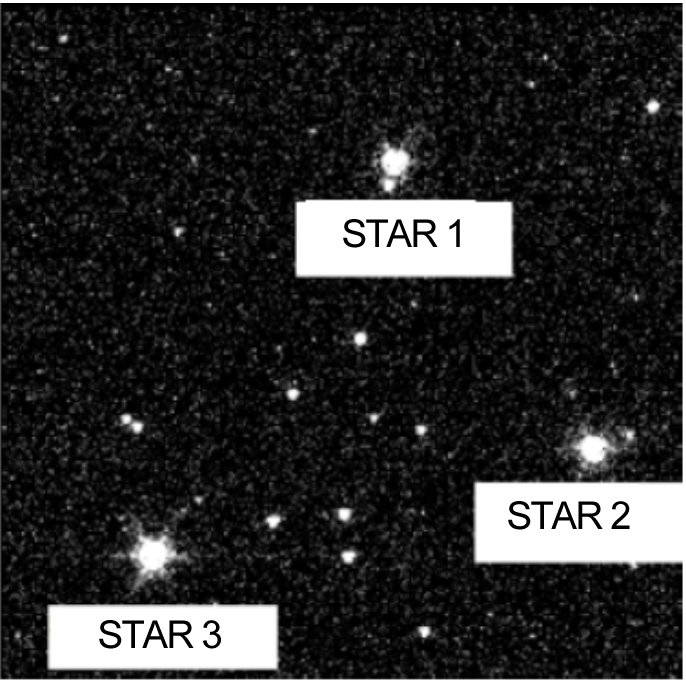## Step 2: Modelling

Draw a picture of a star as seen from Earth, and a planet orbiting that star. Assume that Earth is on the plane of the planet’s orbit around the star.

Note: Instead of a drawing, you can also use a 3D model to represent the star with a light source, a ball for the planet orbiting it and their own eyes being the Earth. When the small ball passes between the student and the light source they should understand that a "little" bit of this light is no longer visible... (actually, the ball should be very small or they must be very far, or the light source must be very large, otherwise the ball will cover all the light source).

Researchers measure the amount of light emitted by the star and received on Earth, over a very long period of time and very regularly. Can this series of measurements show the presence of an exoplanet in orbit around the star?

To find out, sketch the graph of the measured intensity versus time for a star that does not have an exoplanet orbiting it, and for a star that does have an exoplanet orbiting it. Which signal will be observed to prove the presence of an exoplanet?

The student should predict the shape of the two signals: the signal will decrease when the planet passes between the star and the Earth because part of the photons emitted by the star will be blocked by the planet.

You should get a graph that looks like the one presented with the Background information.

Note: For a more advanced level, it can be noticed that if the plane of the orbit is perpendicular to the Earth-star axis, then the planet never passes between the Earth and the star... so this method allows the discovery of a planet only if the Earth-star axis is in the plane of the exoplanet's orbit

## Step 3: Measure

To measure the intensity of the light emitted by the star, researchers use the technique called « photometry ».

To use this technique with the SalsaJ software, open the "Analysis" drop-down menu and choose "Photometry", or click on the photometry icon (see further explanation in "supplementary material").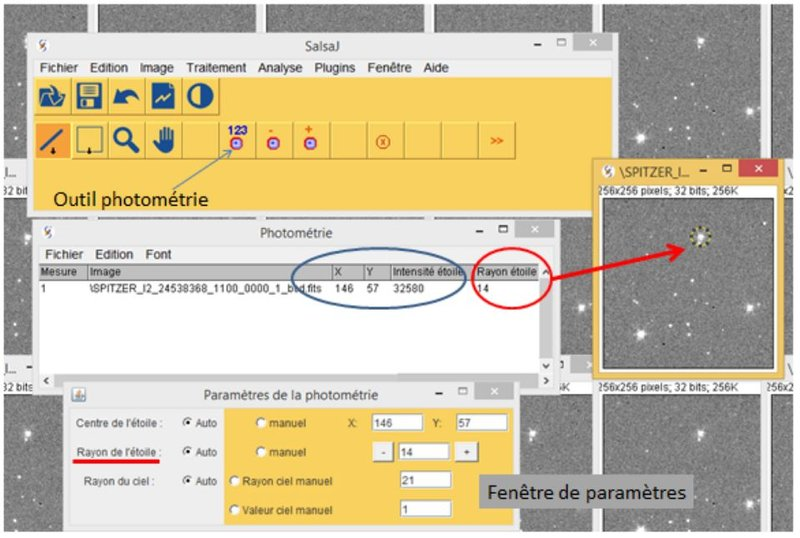Image: Description of the Photometry tool in ©SalsaJ. It includes photometry measures (centre) and parameters (below).

Click on one of the three stars in the first image. A circle appears in the image. The intensity of the star will be calculated within this area (as the integral of the values of all pixels located inside the circle). A new measurement window opens. Here you read the position of the location where you clicked, and the measured intensity.

You can now make measurements on all the images, and for all three stars; you should then transfer these measurements to the spreadsheet provided by the teacher (in the "Raw data" tab) or to a spreadsheet you create yourself (be careful not to mix stars or date...).

Note: if you are able to determine the positions x and y of one star, you may then fix the coordinate in the photometry window. Then, you may click anywhere in the image and the circle will be located at those coordinates. Stars are at the same position in all images (we may be grateful to the researchers who prepared the images in such a nice way…).

## Step 4: Plot

Students will then plot the evolution of the intensity with time for each star. They might do so by making their own spreadsheet and an XY graph by hand. However, drawing curves precise enough to allow an interpretation in terms of size will be very difficult. So, students could use the spreadsheets provided in the attachment, gaining some basic knowledge of spreadsheets before using them.

As soon as data has been pasted in the “Raw data” tab, three sets of data points appear in one graphic in the "Plot and analyses" tab (see additional information for a detailed explanation of the different plots that will be obtained throughout this step).

Students should get a graphic like the one below. The intensity of stars should be as seen in Graph 1 in the image below: Stars 3 > Star 2 > Star 1. Note that the intensity's exact values depend on the parameters chosen for photometry. The position on the image you have clicked on will not have a significant impact.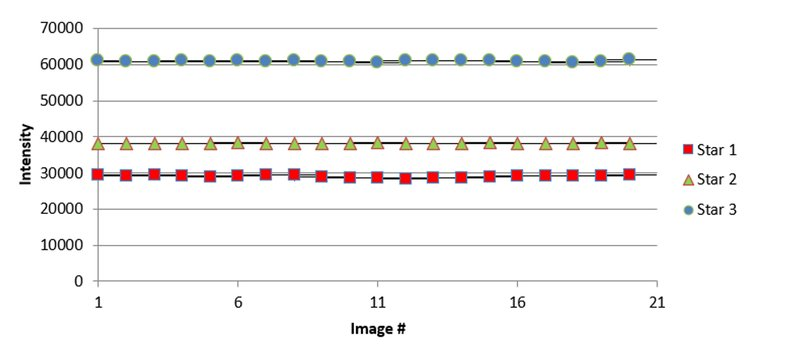Image: graph1.

Looking at the three sets of data (superposed in one graphic) that correspond to three stars, can you observe the effect of an exoplanet for one of the three stars? Not really....

## Step 5: Normalise and interpret

To interpret the plots in Graph1 we will need to make a mathematical operation on the data, which is called “normalisation”.

Have a look at the bottom of the tables in the spreadsheet (column I and line 30).

When the number is set to 0, no operations are made on the data and we obtain Graph1. Try to set the number to “-1” and then to “1”. This will normalise the data in two different ways, and observe how the graphs change.

Can you guess why this is called a “normalisation”? The operation will impose all data to be around the same value - some kind of a “norm”. It gets rid of their difference in terms of average intensity and only focuses on the variation of the intensity. With “1” the data are divided by the average. With “-1” the average is subtracted from the data.

For each normalisation (-1 and 1) we will observe the graphs obtained and ask ourselves if we can observe the effect of an exoplanet on the curve obtained for one of the three stars. Is there a signal that corresponds to what you predicted in step "modelling"?

The image below, Graph2, is the graphic made with a normalisation of “-1”, in which the average has been subtracted from the data. You may guess which star displays a transit, but a negative intensity does not make sense and cannot be described with the model defined in the previous step. Hence, this does not seem to be the best choice.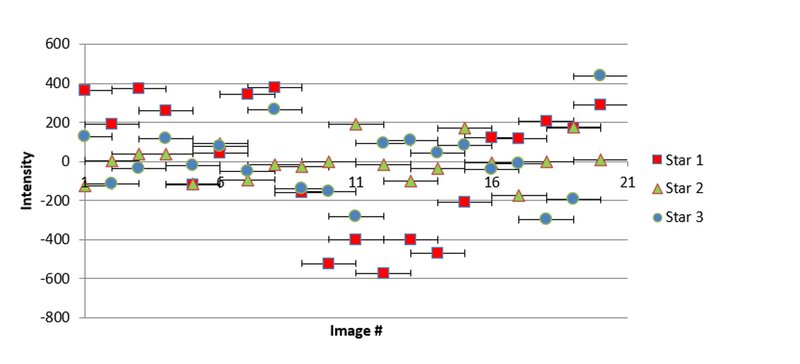Image: graph2 with normalisation -1.

The image below showing Graph3 is made with a normalisation of “1” - data are divided by their average for each set. You want to get the same intensity for all stars as there is no transit, and you want a positive intensity even when there is a transit. So, the best normalisation is obtained with the value set to “1”.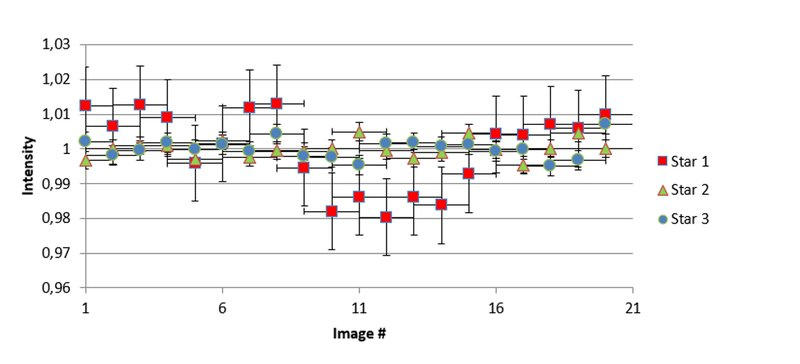Image: graph3 with normalisation 1.

The result is clear from Graph3: STAR number 1 is the one with an exoplanet!

The star you selected through the observation of a special signal is called HD 189733. In October 2005, researchers discovered the presence of the exoplanet HD 189733b orbiting the star using the same method as you just did!

Note: The spreadsheet calculates the dispersion of the measurements and thus plots the error bars. Error bars should decrease as transit dates are correctly identified.

## Step 6: improving measurements (optional)

In Graph 3, the red points are above 1 “outside of the transit” in the graphic. This is not what we predicted in our model. You can find in additional information some indications on how to make the data look better.

Do you want to make the Graph even nicer?

If so, you may specify the dates when you think the transit happens, namely, when the exoplanet covers a portion of the star. To do so, write “1” instead of “0” in column D (called “transit”). Observe how the graphs evolve and get closer to the prediction of your model.

The reason is simple: data points without transit are all around an average of 1. You should get a graphic like the one below - the red points are lowered since the average does not include the data points in the transit anymore. This does not modify other data sets that do not show transits.

During each step of this process, you should observe in the spreadsheet the change in the average intensity for all stars (columns H, I and J), outside (line 1) and within (line 2) the transit. Line 3 indicates the amplitude of the errors (equal to the standard deviation).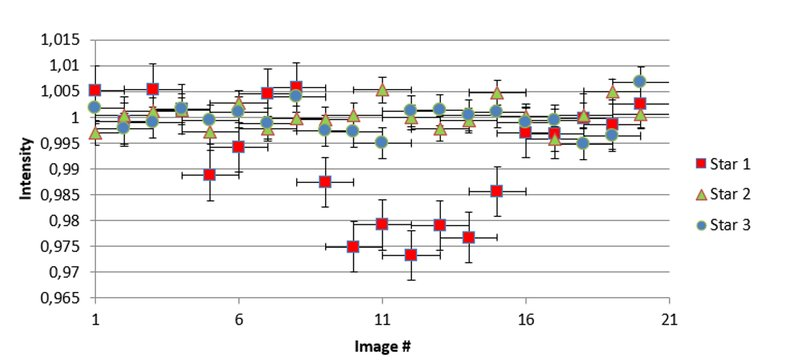Image: graph4.

The result: We have a confirmation that STAR number 1 is the one with an exoplanet!

## Step 7: determine the volume of the exoplanet (optional)

Using the diagram sketched in the first step, can you link the intensity decrease observed in your curve to the physical parameters of the exoplanet HD 189733b and the star HD 189733?

The next questions are propositions to help students find the solution. The answers are given in brackets.

Imagine that all the “little” areas of the star's surface emit the same number of photons.

Let’s imagine that there are 100 photons emitted by one star (for older students, use a letter: there are N photons).

Then, if the surface of the star is twice as large, how many photons should be emitted? (A: twice as many, 200 or 2N). If the surface is 4 times larger? (A: 4 times as many, 400 or 4N). Conclusion: if the surface has an area S, the number of photons is then proportional to the surface S.

Now, if the planet's surface is half of the star, how many photons are blocked during the transit? (A: N/2) If the surface of the planet is smaller, less photons are blocked. So, the number of photons that are blocked is proportional to...? (A: s).

So, the number of photons that are received during the transit is proportional to...? (A: S-s) And if the number is 1 when there is no transit, as in our graphs, then the number during the transit is equals to...? (1-s/S) So, since the number observed during the transit equals 0,98 (use the number that corresponds to what you see on your graph, it should be between 0,97 and 0,98), how many photons have been blocked by the planet? (A: 0,02 - 0,03) So, what is the ratio of the two surfaces? (A: 0,02 - 0,03) And the ratio of the radii? (A: square root of 0,02 or 0,03)

The physical parameters of the star are derived by the observation of the light it emits. Knowing that the radius of the star HD 189733 is equal to 0.805 +/- 0.01 times the radius of the Sun (source: exoplanet.eu), can you deduce the radius of the planet? (uncertainties are given here to emphasize the fact that all measures must be given with an uncertainty. Teachers may not use them with young students - you may also use simple numbers just to get a “rough” idea of the result).

Earth’s radius : RE = 6 370 km

Jupiter’s radius : RJ = 69 900 km ~11 RE

Sun’s radius : R = 696 000 km ~10 RJ

== You should find: radius of HD189733b = 1,138 or 1,137 RJ

You can now check your result on the catalogue of the "Encyclopaedia of extrasolar planets" website http://exoplanet.eu (You will have to write, in the search bar of the catalogue, the following command: "189733" IN NAME)

Note: The radius of the planet equals 1,138
+/- 0,07 RJ in the catalogue. Notice that it was 1,23 RJ in the article published in 2005 and that the estimation of the radius of the star has also changed over the years (see supplementary materials). The quality of observations and models of stars has improved in about 15 years!Evaluation

During or after the activity, students can be asked the following questions (answers between brackets):

• What is an exoplanet? (A planet orbiting a star other than the Sun);
• What is the transit of an exoplanet? (The transit corresponds to the moment when the exoplanet is along the line of sight from Earth to the Star);
• What does photometry measure? (The intensity of the light emitted by a star and collected in the image);
• Sketch the planet / star / Earth model when a transit is observed;
• Sketch the signal that should be observed during the transit;
• If an exoplanet orbits the star that we observe, are we sure to observe the transit? (No, if the signal is not larger than the noise, or if the orbit does not cross the line of sight from Earth to the Star);
• How will the signal change if the exoplanet is bigger? (The signal's intensity will be lower during the transit);
• How will the signal change if the exoplanet moves faster? (If students know about Kepler laws, you may ask if the exoplanet is closer to the star -- the duration of the transit will be shorter).Curriculum

science: spectroscopy and light
science: stars and planets
math: data analysis
math: signal processing (statistics)
math: graphsAdditional Information